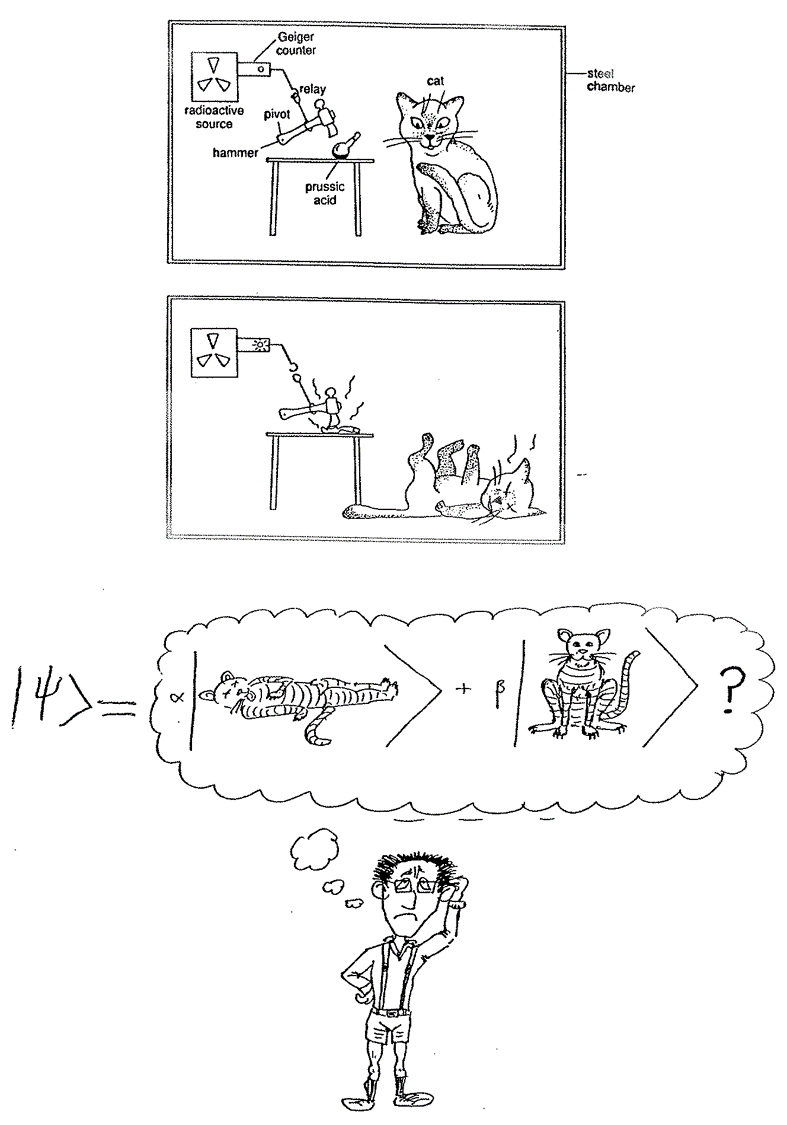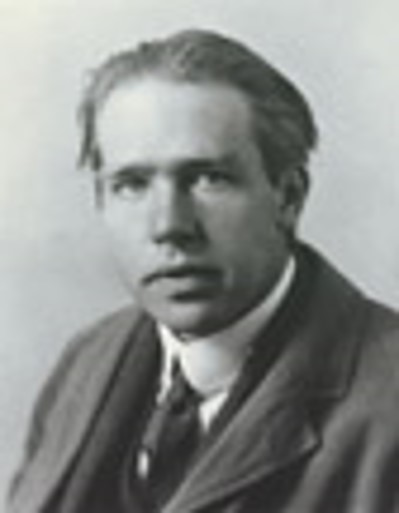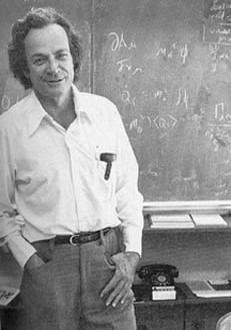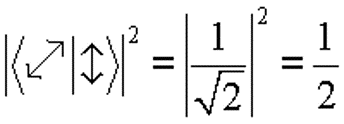# 1.95: What Part of the Quantum Theory Don’t You Understand?

$$\newcommand{\vecs}{\overset { \rightharpoonup} {\mathbf{#1}} }$$ $$\newcommand{\vecd}{\overset{-\!-\!\rightharpoonup}{\vphantom{a}\smash {#1}}}$$$$\newcommand{\id}{\mathrm{id}}$$ $$\newcommand{\Span}{\mathrm{span}}$$ $$\newcommand{\kernel}{\mathrm{null}\,}$$ $$\newcommand{\range}{\mathrm{range}\,}$$ $$\newcommand{\RealPart}{\mathrm{Re}}$$ $$\newcommand{\ImaginaryPart}{\mathrm{Im}}$$ $$\newcommand{\Argument}{\mathrm{Arg}}$$ $$\newcommand{\norm}{\| #1 \|}$$ $$\newcommand{\inner}{\langle #1, #2 \rangle}$$ $$\newcommand{\Span}{\mathrm{span}}$$ $$\newcommand{\id}{\mathrm{id}}$$ $$\newcommand{\Span}{\mathrm{span}}$$ $$\newcommand{\kernel}{\mathrm{null}\,}$$ $$\newcommand{\range}{\mathrm{range}\,}$$ $$\newcommand{\RealPart}{\mathrm{Re}}$$ $$\newcommand{\ImaginaryPart}{\mathrm{Im}}$$ $$\newcommand{\Argument}{\mathrm{Arg}}$$ $$\newcommand{\norm}{\| #1 \|}$$ $$\newcommand{\inner}{\langle #1, #2 \rangle}$$ $$\newcommand{\Span}{\mathrm{span}}$$$$\newcommand{\AA}{\unicode[.8,0]{x212B}}$$

## Quantum Mechanics and Mathematics According to Richard Feynman

• We have come to the conclusion that what are usually called the advanced parts of quantum mechanics are, in fact, quite simple.
• The mathematics that is involved is particularly simple, involving simple algebraic operations and no differential equations or at most very simple ones.Einstein and Schrödinger

## Gott würfelt nicht.## Schrödinger’s CatBohr and Feynman

## Quantum Quotes

• Quantum mechanics is certainly imposing. But an inner voice tells me that it is not yet the real thing. The theory says a lot, but does not really bring us any closer to the secret of the 'old one.' I, at any rate, am convinced that He is not playing at dice. (Einstein)
• I still believe in the possibility of a model of reality, that is to say, of a theory, which represents things themselves and not merely the probability of their occurrence. (Einstein)
• It is wrong to think that the task of physics is to find out how nature is. Physics concerns what we can say about nature. (Bohr)
• Quantum states are states of knowledge and not objective features of the systems they describe. (N. David Mermin)
• I remember discussions with Bohr which went through many hours till very late at night and ended almost in despair; and when at the end of the discussion I went alone for a walk in a neighboring park I repeated to myself again and again the question: Can nature possibly be as absurd as it seemed to us in these atomic experiments? (Heisenberg)

After state preparation by the vertical polarizer, only two subsequent experiments have certain outcomes according to quantum mechanics.

1. The probability that the vertically polarized photons will pass a second vertical polarizer is 1.$$|\langle \updownarrow | \updownarrow \rangle |^{2} = 1$$
2. The probability that the vertically polarized photons will pass a second polarizer that is oriented horizontally is 0.$$|\langle \leftrightarrow| \updownarrow \rangle |^{2} = 0$$

For all other experiments involving two polarizers only the probability of the outcome can be predicted, and this is $$\cos^{2}(\theta)$$, where $$\theta$$ is the relative angle of the polarizing films. For example,## Feynman on the Significance of the Double-Slit Experiment

• We choose to examine a phenomenon which is impossible, absolutely impossible, to explain in any classical way, and which has in it the heart of quantum mechanics.
• In reality, it contains the only mystery.
• We cannot make the mystery go away by “explaining” how it works. We will just tell you how it works.
• In telling you how it works we will have told you about the basic peculiarities of all quantum mechanics.
• Any situation in quantum mechanics can always be explained by saying, “You remember the experiment with the two holes? It’s the same thing.”$|\uparrow\rangle_{S} \stackrel{\mathrm{BS}_{1}}{\longrightarrow} \frac{1}{\sqrt{2}}\left[|\uparrow\rangle_{A}+|\rightarrow\rangle_{B}\right] \nonumber$$|\uparrow\rangle_{S} \stackrel{\mathrm{BS}_{1}}{\longrightarrow} \frac{1}{\sqrt{2}}\left[|\uparrow\rangle_{A}+|\rightarrow\rangle_{B}\right] \nonumber$

$|\uparrow\rangle_{A} \stackrel{\mathrm{BS}_{2}}{\longrightarrow} \frac{1}{\sqrt{2}}\left[|\rightarrow\rangle_{D_{1}}+|\uparrow\rangle_{D_{2}}\right] \qquad |\rightarrow\rangle_{B} \stackrel{\mathrm{BS}_{2}}{\longrightarrow} \frac{1}{\sqrt{2}}\left[|\rightarrow\rangle_{D_{1}}+|\downarrow\rangle_{D_{2}}\right] \nonumber$

$|\uparrow\rangle_{s} \frac{\mathrm{BS}_{1}}{\mathrm{BS}_{2}}>\frac{1}{2}\left[|\rightarrow\rangle_{D_{1}}+|\uparrow\rangle_{D_{2}}+|\rightarrow\rangle_{D_{1}}+|\downarrow\rangle_{D_{2}}\right]=|\rightarrow\rangle_{D_{1}} \nonumber$## Final Quantum Quotes

• If we want to describe what happens in an atomic event, we have to realize that the word “happens” can only apply to the observation, not to the state of affairs between two observations. [Werner Heisenberg]
• I think it is safe to say that no one understands quantum mechanics. Do not keep saying to yourself, if you can possibly avoid it, 'But how can it possibly be like that?' because you will go down the drain into a blind alley from which nobody has yet escaped. Nobody knows how it can be like that. [Richard Feynman]
• Any one who is not shocked by quantum mechanics has not fully understood it. [Niels Bohr]
• The mathematical predictions of quantum mechanics yield results that are in agreement with experimental findings. That is the reason we use quantum theory. That quantum theory fits experiment is what validates the theory, but why experiment should give such peculiar results is a mystery. This is the shock to which Bohr referred. [Marvin Chester with slight modifications by Frank Rioux]
• In the quantum world the present does not always have a unique past.

## Feynman Poetry

We have always had a great deal of difficulty understanding the world view that quantum mechanics represents.

At least I do, because I’m an old enough man that I haven’t got to the point that this stuff is obvious to me.

Okay, I still get nervous with it …

You know how it always is, every new idea, it takes a generation or two until it becomes obvious that there’s no real problem.

I cannot define the real problem, therefore I suspect there’s no real problem, but I’m not sure there’s no real problem.

This page titled 1.95: What Part of the Quantum Theory Don’t You Understand? is shared under a CC BY 4.0 license and was authored, remixed, and/or curated by Frank Rioux via source content that was edited to the style and standards of the LibreTexts platform; a detailed edit history is available upon request.